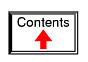# SOLID-STATE DEVICE THEORY

*** INCOMPLETE ***

## Introduction

This chapter will cover the physics behind the operation of semiconductor devices and show how these principles are applied in several different types of semiconductor devices. Subsequent chapters will deal primarily with the practical aspects of these devices in circuits and omit theory as much as possible.

## Quantum physics

"I think it is safe to say that no one understands quantum mechanics."
Physicist Richard P. Feynman

To say that the invention of semiconductor devices was a revolution would not be an exaggeration. Not only was this an impressive technological accomplishment, but it paved the way for developments that would indelibly alter modern society. Semiconductor devices made possible miniaturized electronics, including computers, certain types of medical diagnostic and treatment equipment, and popular telecommunication devices, to name a few applications of this technology.

But behind this revolution in technology stands an even greater revolution in general science: the field of quantum physics. Without this leap in understanding the natural world, the development of semiconductor devices (and more advanced electronic devices still under development) would never have been possible. Quantum physics is an incredibly complicated realm of science, and this chapter is by no means a complete discussion of it, but rather a brief overview. When scientists of Feynman's caliber say that "no one understands [it]," you can be sure it is a complex subject. Without a basic understanding of quantum physics, or at least an understanding of the scientific discoveries that led to its formulation, though, it is impossible to understand how and why semiconductor electronic devices function. Most introductory electronics textbooks I've read attempt to explain semiconductors in terms of "classical" physics, resulting in more confusion than comprehension.

Many of us have seen diagrams of atoms that look something like this: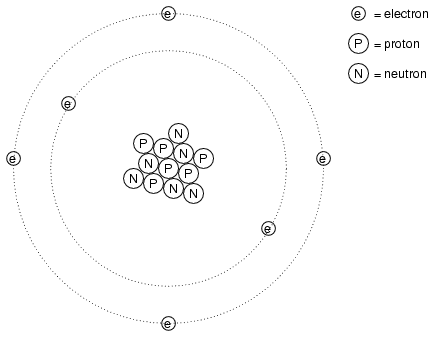Tiny particles of matter called protons and neutrons make up the center of the atom, while electrons orbit around not unlike planets around a star. The nucleus carries a positive electrical charge, owing to the presence of protons (the neutrons have no electrical charge whatsoever), while the atom's balancing negative charge resides in the orbiting electrons. The negative electrons tend to be attracted to the positive protons just as planets are gravitationally attracted toward whatever object(s) they orbit, yet the orbits are stable due to the electrons' motion. We owe this popular model of the atom to the work of Ernest Rutherford, who around the year 1911 experimentally determined that atoms' positive charges were concentrated in a tiny, dense core rather than being spread evenly about the diameter as was proposed by an earlier researcher, J.J. Thompson.

While Rutherford's atomic model accounted for experimental data better than Thompson's, it still wasn't perfect. Further attempts at defining atomic structure were undertaken, and these efforts helped pave the way for the bizarre discoveries of quantum physics. Today our understanding of the atom is quite a bit more complex. However, despite the revolution of quantum physics and the impact it had on our understanding of atomic structure, Rutherford's solar-system picture of the atom embedded itself in the popular conscience to such a degree that it persists in some areas of study even when inappropriate.

Consider this short description of electrons in an atom, taken from a popular electronics textbook:

Orbiting negative electrons are therefore attracted toward the positive nucleus, which leads us to the question of why the electrons do not fly into the atom's nucleus. The answer is that the orbiting electrons remain in their stable orbit due to two equal but opposite forces. The centrifugal outward force exerted on the electrons due to the orbit counteracts the attractive inward force (centripetal) trying to pull the electrons toward the nucleus due to the unlike charges.

In keeping with the Rutherford model, this author casts the electrons as solid chunks of matter engaged in circular orbits, their inward attraction to the oppositely charged nucleus balanced by their motion. The reference to "centrifugal force" is technically incorrect (even for orbiting planets), but is easily forgiven due to its popular acceptance: in reality, there is no such thing as a force pushing any orbiting body away from its center of orbit. It only seems that way because a body's inertia tends to keep it traveling in a straight line, and since an orbit is a constant deviation (acceleration) from straight-line travel, there is constant inertial opposition to whatever force is attracting the body toward the orbit center (centripetal), be it gravity, electrostatic attraction, or even the tension of a mechanical link.

The real problem with this explanation, however, is the idea of electrons traveling in circular orbits in the first place. It is a verifiable fact that accelerating electric charges emit electromagnetic radiation, and this fact was known even in Rutherford's time. Since orbiting motion is a form of acceleration (the orbiting object in constant acceleration away from normal, straight-line motion), electrons in an orbiting state should be throwing off radiation like mud from a spinning tire. Electrons accelerated around circular paths in particle accelerators called synchrotrons are known to do this, and the result is called synchrotron radiation. If electrons were losing energy in this way, their orbits would eventually decay, resulting in collisions with the positively charged nucleus. However, this doesn't ordinarily happen within atoms. Indeed, electron "orbits" are remarkably stable over a wide range of conditions.

Furthermore, experiments with "excited" atoms demonstrated that electromagnetic energy emitted by an atom occurs only at certain, definite frequencies. Atoms that are "excited" by outside influences such as light are known to absorb that energy and return it as electromagnetic waves of very specific frequencies, like a tuning fork that rings at a fixed pitch no matter how it is struck. When the light emitted by an excited atom is divided into its constituent frequencies (colors) by a prism, distinct lines of color appear in the spectrum, the pattern of spectral lines being unique to that element. So regular is this phenomenon that it is commonly used to identify atomic elements, and even measure the proportions of each element in a compound or chemical mixture. According to Rutherford's solar-system atomic model (regarding electrons as chunks of matter free to orbit at any radius) and the laws of classical physics, excited atoms should be able to return energy over a virtually limitless range of frequencies rather than a select few. In other words, if Rutherford's model were correct, there would be no "tuning fork" effect, and the light spectrum emitted by any atom would appear as a continuous band of colors rather than as a few distinct lines.

A pioneering researcher by the name of Neils Bohr attempted to improve upon Rutherford's model after studying in Rutherford's laboratory for several months in 1912. Trying to harmonize the findings of other physicists (most notably, Max Planck and Albert Einstein), Bohr suggested that each electron possessed a certain, specific amount of energy, and that their orbits were likewise quantized such that they could only occupy certain places around the nucleus, somewhat like marbles fixed in circular tracks around the nucleus rather than the free-ranging satellites they were formerly imagined to be. In deference to the laws of electromagnetics and accelerating charges, Bohr referred to these "orbits" as stationary states so as to escape the implication that they were in motion.

While Bohr's ambitious attempt at re-framing the structure of the atom in terms that agreed closer to experimental results was a milestone in physics, it was by no means complete. His mathematical analyses produced better predictions of experimental events than analyses belonging to previous models, but there were still some unanswered questions as to why electrons would behave in such strange ways. The assertion that electrons existed in stationary, quantized states around the nucleus certainly accounted for experimental data better than Rutherford's model, but he had no idea what would force electrons to manifest those particular states. The answer to that question had to come from another physicist, Louis de Broglie, about a decade later.

De Broglie proposed that electrons, like photons (particles of light) manifested both particle-like and wave-like properties. Building on this proposal, he suggested that an analysis of orbiting electrons from a wave perspective rather than a particle perspective might make more sense of their quantized nature. Indeed, this was the case, and another breakthrough in understanding was reached.

The atom according to de Broglie consisted of electrons existing in the form of standing waves, a phenomenon well known to physicists in a variety of forms. Like the plucked string of a musical instrument vibrating at a resonant frequency, with "nodes" and "antinodes" at stable positions along its length, de Broglie envisioned electrons around atoms standing as waves bent around a circle: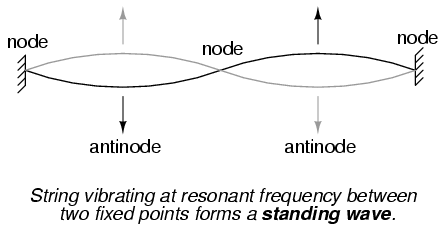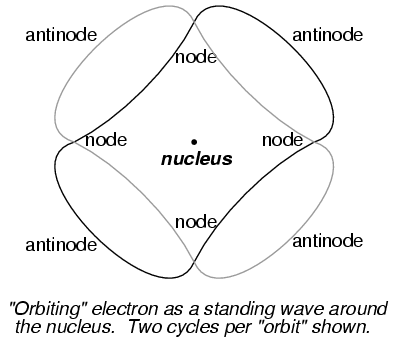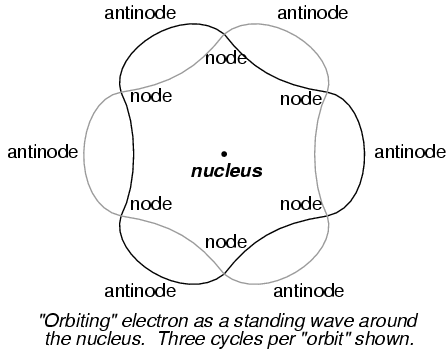Electrons could only exist in certain, definite "orbits" around the nucleus because those were the only distances where the wave ends would match. In any other radius, the wave would destructively interfere with itself and thus cease to exist.

De Broglie's hypothesis gave both mathematical support and a convenient physical analogy to account for the quantized states of electrons within an atom, but his atomic model was still incomplete. Within a few years, though, physicists Werner Heisenberg and Erwin Schrodinger, working independently of each other, built upon de Broglie's concept of a matter-wave duality to create more mathematically rigorous models of subatomic particles.

This theoretical advance from de Broglie's primitive standing wave model to Heisenberg's matrix and Schrodinger's differential equation models was given the name quantum mechanics, and it introduced a rather shocking characteristic to the world of subatomic particles: the trait of probability, or uncertainty. According to the new quantum theory, it was impossible to determine the exact position and exact momentum of a particle at the same time. Popular explanations of this "uncertainty principle" usually cast it in terms of error caused by the process of measurement (i.e. by attempting to precisely measure the position of an electron, you interfere with its momentum and thus cannot know what it was before the position measurement was taken, and visa versa), but the truth is actually much more mysterious than simple measurement interference. The startling implication of quantum mechanics is that particles do not actually possess precise positions and momenta, but rather balance the two quantities in a such way that their combined uncertainties never diminish below a certain minimum value.

It is interesting to note that this form of "uncertainty" relationship exists in areas other than quantum mechanics. As discussed in the "Mixed-Frequency AC Signals" chapter in volume II of this book series, there is a mutually exclusive relationship between the certainty of a waveform's time-domain data and its frequency-domain data. In simple terms, the more precisely we know its constituent frequency(ies), the less precisely we know its amplitude in time, and visa-versa. To quote myself:

A waveform of infinite duration (infinite number of cycles) can be analyzed with absolute precision, but the less cycles available to the computer for analysis, the less precise the analysis. . . The fewer times that a wave cycles, the less certain its frequency is. Taking this concept to its logical extreme, a short pulse -- a waveform that doesn't even complete a cycle -- actually has no frequency, but rather acts as an infinite range of frequencies. This principle is common to all wave-based phenomena, not just AC voltages and currents.

In order to precisely determine the amplitude of a varying signal, we must sample it over a very narrow span of time. However, doing this limits our view of the wave's frequency. Conversely, to determine a wave's frequency with great precision, we must sample it over many, many cycles, which means we lose view of its amplitude at any given moment. Thus, we cannot simultaneously know the instantaneous amplitude and the overall frequency of any wave with unlimited precision. Stranger yet, this uncertainty is much more than observer imprecision; it resides in the very nature of the wave itself. It is not as though it would be possible, given the proper technology, to obtain precise measurements of both instantaneous amplitude and frequency at once. Quite literally, a wave cannot possess both a precise, instantaneous amplitude, and a precise frequency at the same time.

Likewise, the minimum uncertainty of a particle's position and momentum expressed by Heisenberg and Schrodinger has nothing to do with limitation in measurement; rather it is an intrinsic property of the particle's matter-wave dual nature. Electrons, therefore, do not really exist in their "orbits" as precisely defined bits of matter, or even as precisely defined waveshapes, but rather as "clouds" -- the technical term is wavefunction -- of probability distribution, as if each electron were "spread" or "smeared" over a range of positions and momenta.

This radical view of electrons as imprecise clouds at first seems to contradict the original principle of quantized electron states: that electrons exist in discrete, defined "orbits" around atomic nuclei. It was, after all, this discovery that led to the formation of quantum theory to explain it. How odd it seems that a theory developed to explain the discrete behavior of electrons ends up declaring that electrons exist as "clouds" rather than as discrete pieces of matter. However, the quantized behavior of electrons does not depend on electrons having definite position and momentum values, but rather on other properties called quantum numbers. In essence, quantum mechanics dispenses with commonly held notions of absolute position and absolute momentum, and replaces them with absolute notions of a sort having no analogue in common experience.

Even though electrons are known to exist in ethereal, "cloud-like" forms of distributed probability rather than as discrete chunks of matter, those "clouds" possess other characteristics that are discrete. Any electron in an atom can be described in terms of four numerical measures (the previously mentioned quantum numbers), called the Principal, Angular Momentum, Magnetic, and Spin numbers. The following is a synopsis of each of these numbers' meanings:

Principal Quantum Number: Symbolized by the letter n, this number describes the shell that an electron resides in. An electron "shell" is a region of space around an atom's nucleus that electrons are allowed to exist in, corresponding to the stable "standing wave" patterns of de Broglie and Bohr. Electrons may "leap" from shell to shell, but cannot exist between the shell regions.

The principle quantum number can be any positive integer (a whole number, greater than or equal to 1). In other words, there is no such thing as a principle quantum number for an electron of 1/2 or -3. These integer values were not arrived at arbitrarily, but rather through experimental evidence of light spectra: the differing frequencies (colors) of light emitted by excited hydrogen atoms follow a sequence mathematically dependent on specific, integer values.

Each shell has the capacity to hold multiple electrons. An analogy for electron shells is the concentric rows of seats of an amphitheater. Just as a person seated in an amphitheater must choose a row to sit in (for there is no place to sit in the space between rows), electrons must "choose" a particular shell to "sit" in. Like amphitheater rows, the outermost shells are able to hold more electrons than the inner shells. Also, electrons tend to seek the lowest available shell, like people in an amphitheater trying to find the closest seat to the center stage. The higher the shell number, the greater the energy of the electrons in it.

The maximum number of electrons that any shell can hold is described by the equation 2n2, where "n" is the principle quantum number. Thus, the first shell (n=1) can hold 2 electrons; the second shell (n=2) 8 electrons, and the third shell (n=3) 18 electrons.

Electron shells in an atom are sometimes designated by letter rather than by number. The first shell (n=1) is labeled K, the second shell (n=2) L, the third shell (n=3) M, the fourth shell (n=4) N, the fifth shell (n=5) O, the sixth shell (n=6) P, and the seventh shell (n=7) Q.

Angular Momentum Quantum Number: Within each shell, there are subshells. One might be inclined to think of subshells as simple subdivisions of shells, like lanes dividing a road, but the truth is much stranger than this. Subshells are regions of space where electron "clouds" are allowed to exist, and different subshells actually have different shapes. The first subshell is shaped like a sphere, which makes sense to most people, visualizing a cloud of electrons surrounding the atomic nucleus in three dimensions. The second subshell, however, resembles a dumbbell, comprised of two "lobes" joined together at a single point near the atom's center. The third subshell typically resembles a set of four "lobes" clustered around the atom's nucleus. These subshell shapes are reminiscent of graphical depictions of radio antenna signal strength, with bulbous lobe-shaped regions extending from the antenna in various directions.

Valid angular momentum quantum numbers are positive integers like principal quantum numbers, but also include zero. These quantum numbers for electrons are symbolized by the letter l. The number of subshells in a shell is equal to the shell's principal quantum number. Thus, the first shell (n=1) has one subshell, numbered 0; the second shell (n=2) has two subshells, numbered 0 and 1; the third shell (n=3) has three subshells, numbered 0, 1, and 2.

An older convention for subshell description used letters rather than numbers. In this notational system, the first subshell (l=0) was designated s, the second subshell (l=1) designated p, the third subshell (l=2) designated d, and the fourth subshell (l=3) designated f. The letters come from the words sharp, principal (not to be confused with the principal quantum number, n), diffuse, and fundamental. You will still see this notational convention in many periodic tables, used to designate the electron configuration of the atoms' outermost, or valence, shells.

Magnetic Quantum Number: The magnetic quantum number for an electron classifies which orientation its subshell shape is pointed. For each subshell in each shell, there are multiple directions in which the "lobes" can point, and these different orientations are called orbitals. For the first subshell (s; l=0), which resembles a sphere, there is no "direction" it can "point," so there is only one orbital. For the second (p; l=1) subshell in each shell, which resembles a dumbbell, there are three different directions they can be oriented (think of three dumbbells intersecting in the middle, each oriented along a different axis in a three-axis coordinate system).

Valid numerical values for this quantum number consist of integers ranging from -l to l, and are symbolized as ml in atomic physics and lz in nuclear physics. To calculate the number of orbitals in any given subshell, double the subshell number and add 1 (2l + 1). For example, the first subshell (l=0) in any shell contains a single orbital, numbered 0; the second subshell (l=1) in any shell contains three orbitals, numbered -1, 0, and 1; the third subshell (l=2) contains five orbitals, numbered -2, -1, 0, 1, and 2; and so on.

Like principal quantum numbers, the magnetic quantum number arose directly from experimental evidence: the division of spectral lines as a result of exposing an ionized gas to a magnetic field, hence the name "magnetic" quantum number.

Spin Quantum Number: Like the magnetic quantum number, this property of atomic electrons was discovered through experimentation. Close observation of spectral lines revealed that each line was actually a pair of very closely-spaced lines, and this so-called fine structure was hypothesized to be the result of each electron "spinning" on an axis like a planet. Electrons with different "spins" would give off slightly different frequencies of light when excited, and so the quantum number of "spin" came to be named as such. The concept of a spinning electron is now obsolete, being better suited to the (incorrect) view of electrons as discrete chunks of matter rather than as the "clouds" they really are, but the name remains.

Spin quantum numbers are symbolized as ms in atomic physics and sz in nuclear physics. For each orbital in each subshell in each shell, there can be two electrons, one with a spin of +1/2 and the other with a spin of -1/2.

The physicist Wolfgang Pauli developed a principle explaining the ordering of electrons in an atom according to these quantum numbers. His principle, called the Pauli exclusion principle, states that no two electrons in the same atom may occupy the exact same quantum states. That is, each electron in an atom has a unique set of quantum numbers. This limits the number of electrons that may occupy any given orbital, subshell, and shell.

Shown here is the electron arrangement for a hydrogen atom: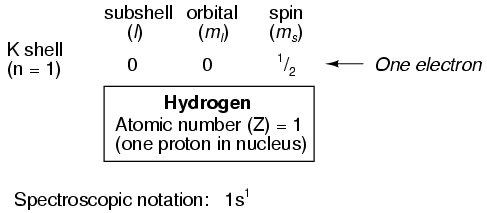With one proton in the nucleus, it takes one electron to electrostatically balance the atom (the proton's positive electric charge exactly balanced by the electron's negative electric charge). This one electron resides in the lowest shell (n=1), the first subshell (l=0), in the only orbital (spatial orientation) of that subshell (ml=0), with a spin value of 1/2. A very common method of describing this organization is by listing the electrons according to their shells and subshells in a convention called spectroscopic notation. In this notation, the shell number is shown as an integer, the subshell as a letter (s,p,d,f), and the total number of electrons in the subshell (all orbitals, all spins) as a superscript. Thus, hydrogen, with its lone electron residing in the base level, would be described as 1s1.

Proceeding to the next atom type (in order of atomic number), we have the element helium: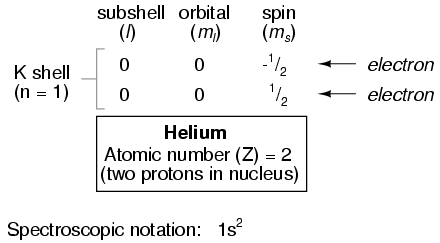A helium atom has two protons in the nucleus, and this necessitates two electrons to balance the double-positive electric charge. Since two electrons -- one with spin=1/2 and the other with spin=-1/2 -- will fit into one orbital, the electron configuration of helium requires no additional subshells or shells to hold the second electron.

However, an atom requiring three or more electrons will require additional subshells to hold all electrons, since only two electrons will fit into the lowest shell (n=1). Consider the next atom in the sequence of increasing atomic numbers, lithium: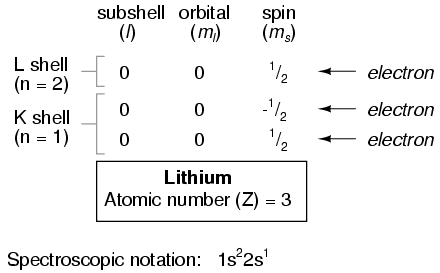An atom of lithium only uses a fraction of the L shell's (n=2) capacity. This shell actually has a total capacity of eight electrons (maximum shell capacity = 2n2 electrons). If we examine the organization of the atom with a completely filled L shell, we will see how all combinations of subshells, orbitals, and spins are occupied by electrons: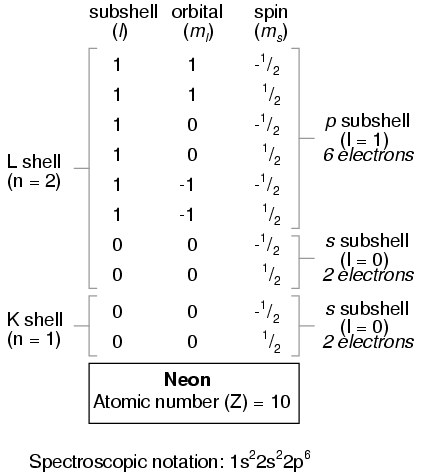Often, when the spectroscopic notation is given for an atom, any shells that are completely filled are omitted, and only the unfilled, or the highest-level filled shell, is denoted. For example, the element neon (shown in the previous illustration), which has two completely filled shells, may be spectroscopically described simply as 2p6 rather than 1s22s22p6. Lithium, with its K shell completely filled and a solitary electron in the L shell, may be described simply as 2s1 rather than 1s22s1.

The omission of completely filled, lower-level shells is not just a notational convenience. It also illustrates a basic principle of chemistry: that the chemical behavior of an element is primarily determined by its unfilled shells. Both hydrogen and lithium have a single electron in their outermost shells (1s1 and 2s1, respectively), and this gives the two elements some similar properties. Both are highly reactive, and reactive in much the same way (bonding to similar elements in similar modes). It matters little that lithium has a completely filled K shell underneath its almost-vacant L shell: the unfilled L shell is the shell that determines its chemical behavior.

Elements having completely filled outer shells are classified as noble, and are distinguished by their almost complete non-reactivity with other elements. These elements used to be classified as inert, when it was thought that they were completely unreactive, but it is now known that they may form compounds with other elements under certain conditions.

Given the fact that elements with identical electron configurations in their outermost shell(s) exhibit similar chemical properties, it makes sense to organize the different elements in a table accordingly. Such a table is known as a periodic table of the elements, and modern tables follow this general form: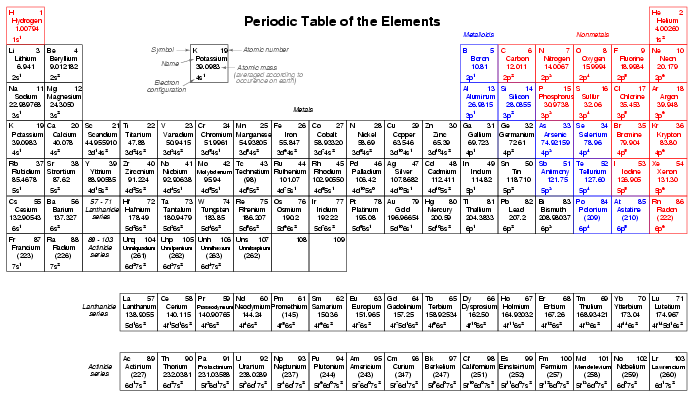Dmitri Mendeleev, a Russian chemist, was the first to develop a periodic table of the elements. Although Mendeleev organized his table according to atomic mass rather than atomic number, and so produced a table that was not quite as useful as modern periodic tables, his development stands as an excellent example of scientific proof. Seeing the patterns of periodicity (similar chemical properties according to atomic mass), Mendeleev hypothesized that all elements would fit into this ordered scheme. When he discovered "empty" spots in the table, he followed the logic of the existing order and hypothesized the existence of heretofore undiscovered elements. The subsequent discovery of those elements granted scientific legitimacy to Mendeleev's hypothesis, further discoveries leading to the form of the periodic table we use today.

This is how science should work: hypotheses followed to their logical conclusions, and accepted, modified, or rejected as determined by the agreement of experimental data to those conclusions. Any fool can formulate a hypothesis after-the-fact to explain existing experimental data, and many do. What sets a scientific hypothesis apart from post hoc speculation is the prediction of future experimental data yet uncollected, and the possibility of disproof as a result of that data. To boldly follow a hypothesis to its logical conclusion(s) and dare to predict the results of future experiments is not a dogmatic leap of faith, but rather a public test of that hypothesis, open to challenge from anyone able to produce contradictory data. In other words, scientific hypotheses are always "risky" in the sense that they claim to predict the results of experiments not yet conducted, and are therefore susceptible to disproof if the experiments do not turn out as predicted. Thus, if a hypothesis successfully predicts the results of repeated experiments, there is little probability of its falsehood.

Quantum mechanics, first as a hypothesis and later as a theory, has proven to be extremely successful in predicting experimental results, hence the high degree of scientific confidence placed in it. Many scientists have reason to believe that it is an incomplete theory, though, as its predictions hold true more so at very small physical scales than at macroscopic dimensions, but nevertheless it is a tremendously useful theory in explaining and predicting the interactions of particles and atoms.

As you have already seen in this chapter, quantum physics is essential in describing and predicting many different phenomena. In the next section, we will see its significance in the electrical conductivity of solid substances, including semiconductors. Simply put, nothing in chemistry or solid-state physics makes sense within the popular theoretical framework of electrons existing as discrete chunks of matter, whirling around atomic nuclei like miniature satellites. It is only when electrons are viewed as "wavefunctions" existing in definite, discrete states that the regular and periodic behavior of matter can be explained.

• REVIEW:
• Electrons in atoms exist in "clouds" of distributed probability, not as discrete chunks of matter orbiting the nucleus like tiny satellites, as common illustrations of atoms show.
• Individual electrons around an atomic nucleus seek unique "states," described by four quantum numbers: the Principal Quantum Number, otherwise known as the shell; the Angular Momentum Quantum Number, otherwise known as the subshell; the Magnetic Quantum Number, describing the orbital (subshell orientation); and the Spin Quantum Number, or simply spin. These states are quantized, meaning that there are no "in-between" conditions for an electron other than those states that fit into the quantum numbering scheme.
• The Principal Quantum Number (n) describes the basic level or shell that an electron resides in. The larger this number, the greater radius the electron cloud has from the atom's nucleus, and the greater than electron's energy. Principal quantum numbers are whole numbers (positive integers).
• The Angular Momentum Quantum Number (l) describes the shape of the electron cloud within a particular shell or level, and is often known as the "subshell." There are as many subshells (electron cloud shapes) in any given shell as that shell's principal quantum number. Angular momentum quantum numbers are positive integers beginning at zero and terminating at one less than the principal quantum number (n-1).
• The Magnetic Quantum Number (ml) describes which orientation a subshell (electron cloud shape) has. There are as many different orientations for each subshell as the subshell number (l) plus 1, and each unique orientation is called an orbital. These numbers are integers ranging from the negative value of the subshell number (l) through 0 to the positive value of the subshell number.
• The Spin Quantum Number (ms) describes another property of an electron, and can be a value of +1/2 or -1/2.
• Pauli's Exclusion Principle says that no two electrons in an atom may share the exact same set of quantum numbers. Therefore, there is room for two electrons in each orbital (spin=1/2 and spin=-1/2), 2l+1 orbitals in every subshell, and n subshells in every shell, and no more.
• Spectroscopic notation is a convention for denoting the electron configuration of an atom. Shells are shown as whole numbers, followed by subshell letters (s,p,d,f), with superscripted numbers totaling the number of electrons residing in each respective subshell.
• An atom's chemical behavior is solely determined by the electrons in the unfilled shells. Low-level shells that are completely filled have little or no effect on the chemical bonding characteristics of elements.
• Elements with completely filled electron shells are almost entirely unreactive, and are called noble (formerly known as inert).

## Band theory of solids

Quantum physics describes the states of electrons in an atom according to the four-fold scheme of quantum numbers. The quantum number system describes the allowable states electrons may assume in an atom. To use the analogy of an amphitheater, quantum numbers describe how many rows and seats there are. Individual electrons may be described by the combination of quantum numbers they possess, like a spectator in an amphitheater assigned to a particular row and seat.

Like spectators in an amphitheater moving between seats and/or rows, electrons may change their statuses, given the presence of available spaces for them to fit, and available energy. Since shell level is closely related to the amount of energy that an electron possesses, "leaps" between shell (and even subshell) levels requires transfers of energy. If an electron is to move into a higher-order shell, it requires that additional energy be given to the electron from an external source. Using the amphitheater analogy, it takes an increase in energy for a person to move into a higher row of seats, because that person must climb to a greater height against the force of gravity. Conversely, an electron "leaping" into a lower shell gives up some of its energy, like a person jumping down into a lower row of seats, the expended energy manifesting as heat and sound released upon impact.

Not all "leaps" are equal. Leaps between different shells requires a substantial exchange of energy, while leaps between subshells or between orbitals require lesser exchanges.

When atoms combine to form substances, the outermost shells, subshells, and orbitals merge, providing a greater number of available energy levels for electrons to assume. When large numbers of atoms exist in close proximity to each other, these available energy levels form a nearly continuous band wherein electrons may transition.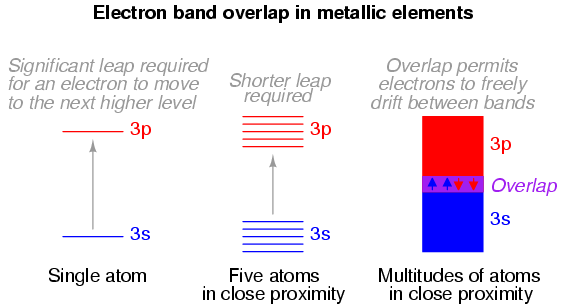It is the width of these bands and their proximity to existing electrons that determines how mobile those electrons will be when exposed to an electric field. In metallic substances, empty bands overlap with bands containing electrons, meaning that electrons may move to what would normally be (in the case of a single atom) a higher-level state with little or no additional energy imparted. Thus, the outer electrons are said to be "free," and ready to move at the beckoning of an electric field.

Band overlap will not occur in all substances, no matter how many atoms are in close proximity to each other. In some substances, a substantial gap remains between the highest band containing electrons (the so-called valence band) and the next band, which is empty (the so-called conduction band). As a result, valence electrons are "bound" to their constituent atoms and cannot become mobile within the substance without a significant amount of imparted energy. These substances are electrical insulators: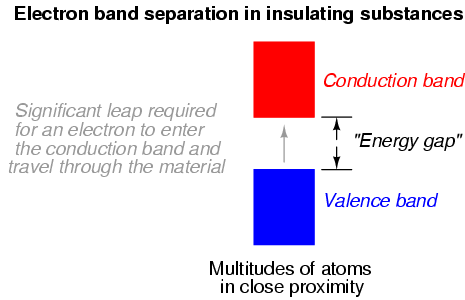Materials that fall within the category of semiconductors have a narrow gap between the valence and conduction bands. Thus, the amount of energy required to motivate a valence electron into the conduction band where it becomes mobile is quite modest: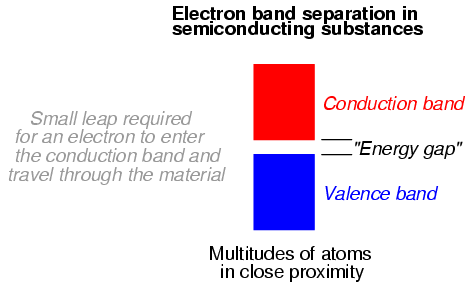At low temperatures, there is little thermal energy available to push valence electrons across this gap, and the semiconducting material acts as an insulator. At higher temperatures, though, the ambient thermal energy becomes sufficient to force electrons across the gap, and the material will conduct electricity.

It is difficult to predict the conductive properties of a substance by examining the electron configurations of its constituent atoms. While it is true that the best metallic conductors of electricity (silver, copper, and gold) all have outer s subshells with a single electron, the relationship between conductivity and valence electron count is not necessarily consistent: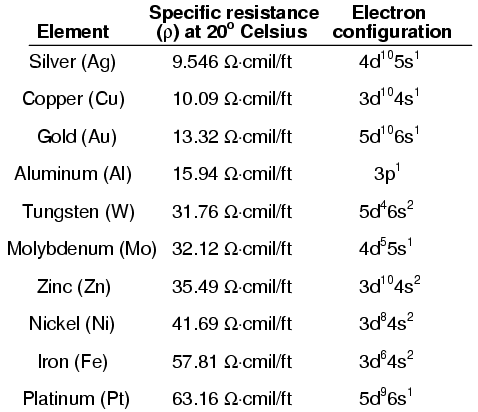Likewise, the electron band configurations produced by compounds of different elements defies easy association with the electron configurations of its constituent elements.

• REVIEW:

• REVIEW:

• REVIEW:

## Junction diodes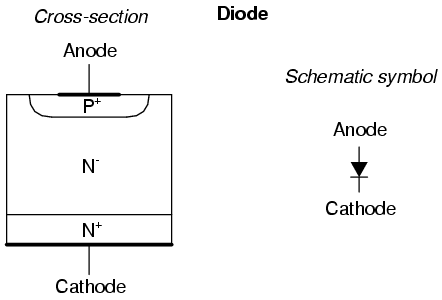• REVIEW:

## Bipolar junction transistors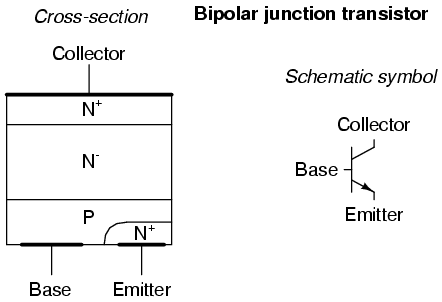• REVIEW:

## Junction field-effect transistors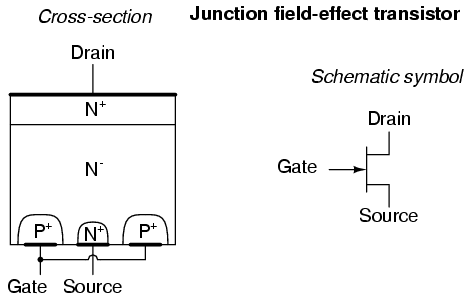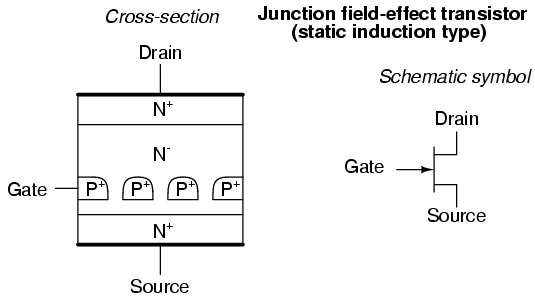• REVIEW:

## Insulated-gate field-effect transistors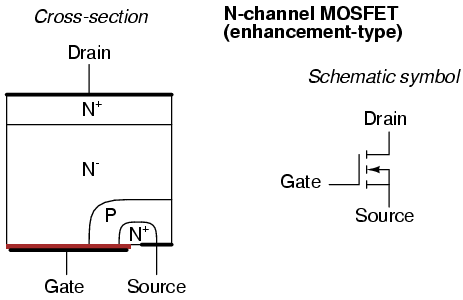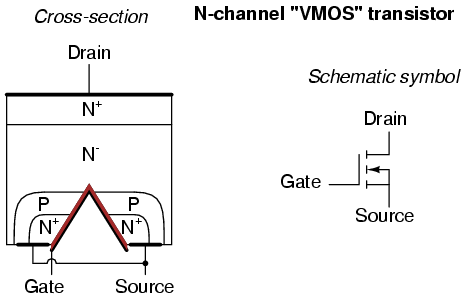• REVIEW:

## Thyristors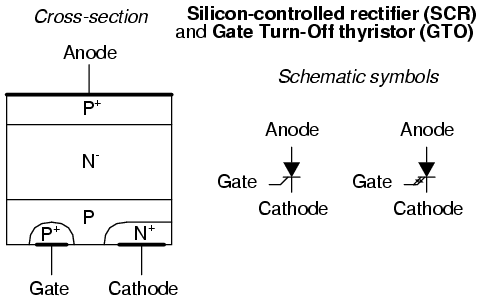• REVIEW:

• REVIEW:

• REVIEW:

• REVIEW:

• REVIEW:

## Contributors

Contributors to this chapter are listed in chronological order of their contributions, from most recent to first. See Appendix 2 (Contributor List) for dates and contact information.

Bill Heath (September 2002): Pointed out error in illustration of carbon atom -- the nucleus was shown with seven protons instead of six.

Lessons In Electric Circuits copyright (C) 2000-2003 Tony R. Kuphaldt, under the terms and conditions of the Design Science License.